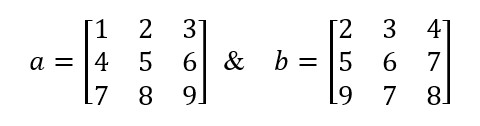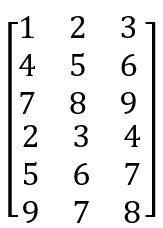# Problem 55485. Array Concatenation (2)

Given two matrices, a and b, concatenate the two matrices vertically, i.e., the number of rows of the result should be equal to the sum of the number of rows of matrix a and matrix b. Assume both matrices a and b have the the same number of columns and the result will also have the same number of columns.
For example, ifThe result should be### Solution Stats

83.13% Correct | 16.87% Incorrect
Last Solution submitted on Dec 01, 2023

### Community Treasure Hunt

Find the treasures in MATLAB Central and discover how the community can help you!

Start Hunting!